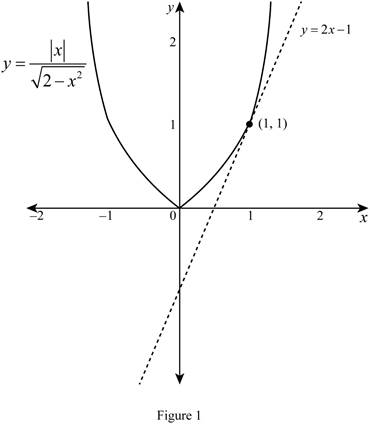# The equation of the tangent line to the curve at the point.### Single Variable Calculus: Concepts...

4th Edition
James Stewart
Publisher: Cengage Learning
ISBN: 9781337687805### Single Variable Calculus: Concepts...

4th Edition
James Stewart
Publisher: Cengage Learning
ISBN: 9781337687805

#### Solutions

Chapter 3.4, Problem 46E

(a)

To determine

## To find: The equation of the tangent line to the curve at the point.

Expert Solution

The equation of the tangent line to the curve y=|x|2x2 at (1,1) is y=2x1_.

### Explanation of Solution

Given:

The function is y=|x|2x2 and the point is (1,1).

Result used:

The Power Rule combined with the Chain Rule:

If n is any real number and g(x) is differentiable function, then

ddx[g(x)]n=n[g(x)]n1g(x) (1)

Quotient Rule:

If f(x). and g(x) are both differentiable function, then

ddx[f(x)g(x)]=g(x)ddx[f(x)]f(x)ddx[g(x)][g(x)]2 (2)

Formula used:

The equation of the tangent line at (x1,y1) is, yy1=m(xx1) (3)

where, m is the slope of the tangent line at (x1,y1) and m=dydx|x=x1.

Calculation:

For x>0,|x|=x and f(x)=x2x2.

The derivative of y is dydx, which is obtained as follows,

dydx=ddx(y)=ddx(|x|2x2)=ddx(x2x2)

Apply the quotient rule as shown in equation (2),

dydx=2x2ddx[x]xddx[2x2][2x2]2

Apply the power rule combined with the chain rule as shown in equation (1),

dydx=2x2[1x11]xddx(2x2)12[(2x2)12]2=2x2x12(2x2)121ddx(2x2)(2x2)=2x2x12(2x2)122(ddx(2)ddx(x2))(2x2)=2x2x12(2x2)12(02x21)(2x2)

On further simplification, the derivative of the function becomes,

dydx=2x2+x2(2x2)12(2x)(2x2)=2x2+x22x2(2x2)=2x2+x22x2(2x2)=22x2(2x2)

Therefore, the derivative of y=|x|2x2 is dydx=22x2(2x2)_.

The slope of the tangent line at (1,1) is computed as follows,

m=dydx|x=1=22(1)2(2(1)2)    =21(1)  =2

Thus, the slope of the tangent line is m=2.

Substitute (1,1) for (x1,y1) and 2 for m in equation (1),

y1=2(x1)y1=2x2y=2x2+1y=2x1

Therefore, the equation of the tangent line is y=2x1.

(b)

To determine

Expert Solution

### Explanation of Solution

Given:

The equation of the curve is y=|x|2x2.

The equation of the tangent line is y=2x1.

Graph:

Use the online graphing calculator to draw the graph of the functions as shown below in Figure 1.From Figure 1, it is observed that the equation of the tangent line touches on the curve y=|x|2x2 at the point (1,1).

### Have a homework question?

Subscribe to bartleby learn! Ask subject matter experts 30 homework questions each month. Plus, you’ll have access to millions of step-by-step textbook answers!